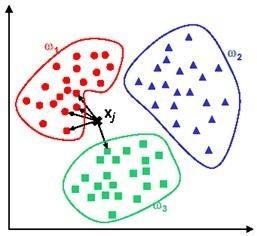# kNN vs k-Means

k-NN

Classification algorithm, and is a subset of supervised learning. k-NN is for number of nearest neighbors. For example, you already have a set of identified clusters groups and you have a new point added to your data set. Thus, you want to know which cluster your new point belongs to. Therefore, you see how many neighbors your new point has in which cluster.For example, if you have a 5-NN as in 5 nearest neighbors, it detects that out of the 5 closest neighbours, 4 are in $\omega_1$ and 1 are in $\omega_3$, therefore your new point belongs to $\omega_1$.

k-Means

Clustering algorithm, and is a subset of unsupervised learning. $k$ refers to the defined number of clusters for the algorithm. K-means is a family of moving centroid algorithms, such that at every iteration the center of the cluster moves slightly to minimize the objective function. This continues until (i) the means stop changing (ii) the maximum nunber of iterations has been reached.